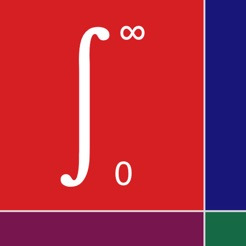Screenshots

•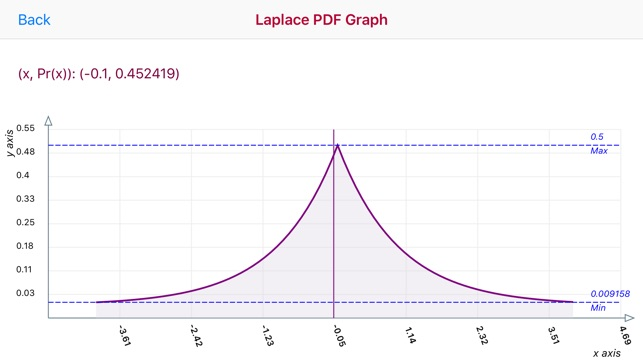•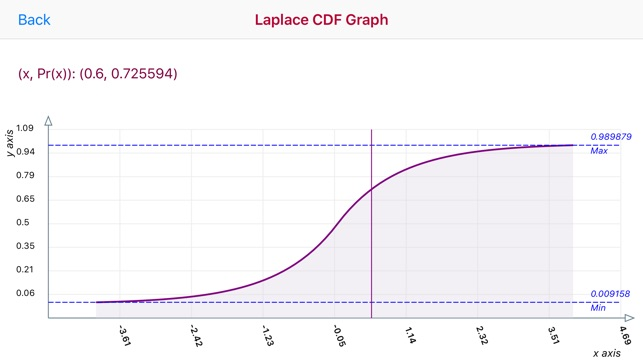•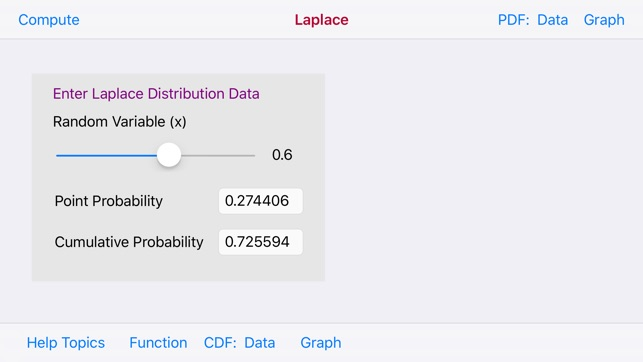•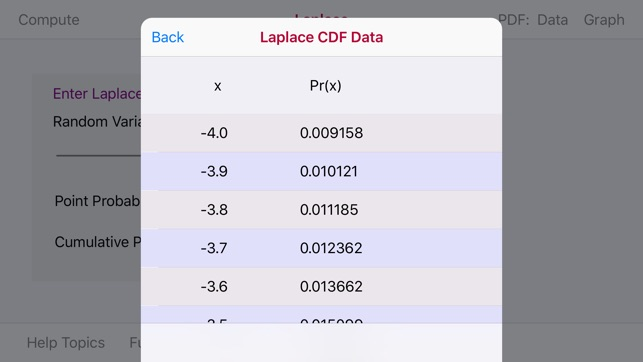•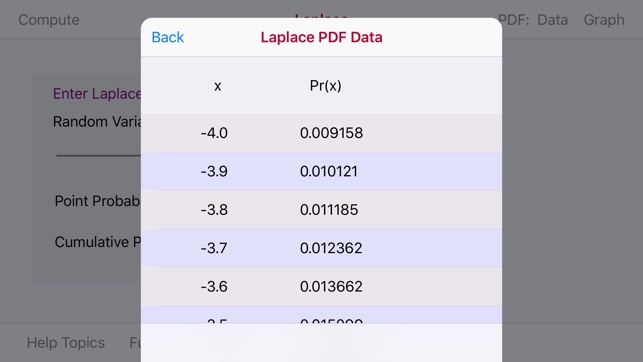Description

In probability theory and statistics, the Laplace Distribution is a continuous probability distribution which is the distribution of differences between two independent variates with identical exponential distributions.

The Laplace Distribution app provides for the data entry of one variable: a Random Variable (x) The range for the Random Variable (x) is -4.0 - 4.0. Data Entry is accomplished via a Data Slider

The Laplace Distribution app produces a PDF/CDF Graph and PDF/CDF Data Tables The Random Variable (x) selected is used to compute the PDF/CDF data.

The PDF and CDF graphs are touch interactive graphs for computed (x/Pr(x) paired values. The graphs hav a touch feature whereby upon the touch a slidable vertical line appears. Upon movement of the line a paired (x,Pr(x) values appear relative to the line position on the graph curve.

The horizontal x-axis displays computed (x) values. The vertical y-axis plots a range of Pr(X) values.

The Laplace Apple Watch Extension provides for a key entry of the Random Variable (x) with a range of -4.0 to 4.0 and displays the Point and Cumulative Probabilities

Version 1.1

Information

Seller
Donald Schaefer
Size
7.9 MB
Category
Education
Compatibility

Requires iOS 12.0 or later. Compatible with iPhone, iPad, and iPod touch.

Languages

English

Age Rating
Rated 4+
Price
Free

Supports

•